Nonlinear Equations¶

The methods for solving nonlinear equations can be subdivided into single versus multivariate case. Good starting points for learning about how to solve nonlinear equation using SciPy are the tutorial and reference pages of the scipy.optimize package.

One variable root finding¶

For single variable case there are slower methods with guarantees such as interval bisection and faster methods that need a good starting guess such as Newton's method. In modern software the good properties of the two have been combined. The best method to try is probably Brent's method implemented in scipy.optimize.brentq. Other methods such as Newton's method are also available in optimize package. The methods available will typically find some root, not all. In that sense the methods are local.

In this example we will find a root of $x^3 - x^2 -1$. It is always good to combine root finding with visualizing the function to get an idea of the starting points or intervals of interest.

In :
%matplotlib inline

In :
from scipy.optimize import brentq
import matplotlib.pyplot as plt

def f(x):
return x**3 - x**2 - 1

x = linspace(0, 2, 100)
plt.plot(x, f(x))
plt.axhline(color = 'k')
root, info = brentq(f, 0.5, 2, full_output=True)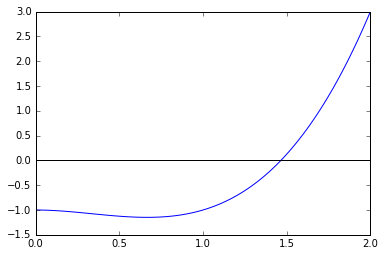In :
print root, info.converged

1.46557123188 True

In :
plt.plot(x, f(x))
plt.axhline(color = 'k')
plt.axvline(x=root, color = 'r', **{'linestyle': 'dashed'})

Out:
<matplotlib.lines.Line2D at 0x10658f710>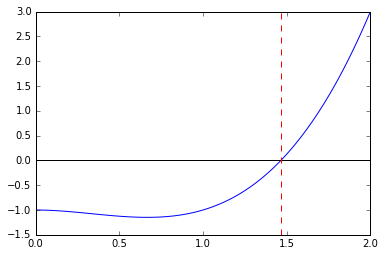Here is another example, this time for the function $(x^2 \sin(20 x) + \cos(x - \pi))^3 + 1$. Lets say we are interested in finding the root near 0 where the function is somewhat flat.

In :
from math import pi
from numpy import sin, cos, linspace

def g(x):
return (x**2. * sin(20*x) + cos(x-pi))**3 + 1

x = linspace(-1, 1, 100)
plt.plot(x, g(x))
plt.show()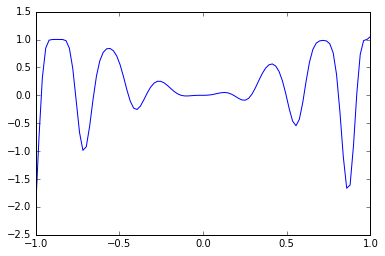We can zoom in to get a better idea of the interval of interest.

In :
x = linspace(-0.05, 0.05, 100)
plt.plot(x, g(x))
plt.show()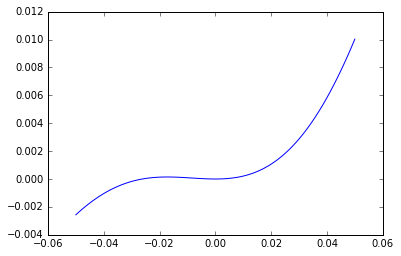In :
x = linspace(-0.04, -0.02, 50)
plt.plot(x, g(x))
plt.show()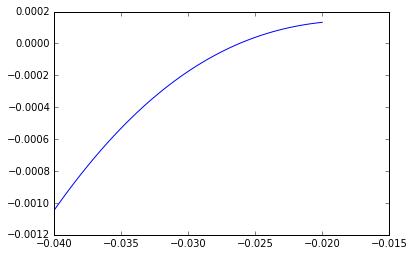In :
root = brentq(g, -0.03, -0.02)
print root
print g(root)

-0.0261782902512
-1.59872115546e-14


Solving a system of nonlinear equations¶

In :
from numpy import array
from scipy.optimize import root

def function(x):
f = array([x + 2.* x - 2, x**2 + 4. * x**2 - 4])
df = array([[1., 2], [2 * x, 8 * x]])
return f, df

sol = root(function, array([1., 2]), method='hybr', jac = True)

In :
print sol

    fjac: array([[-0.55009815, -0.83510001],
[ 0.83510001, -0.55009815]])
fun: array([ 0.,  0.])
message: 'The solution converged.'
nfev: 11
njev: 1
qtf: array([  9.48657074e-12,   6.24900607e-12])
r: array([ -1.81785741, -10.31651456,  -4.40078498])
status: 1
success: True
x: array([ -5.50632813e-18,   1.00000000e+00])

In :
print function(sol.x)

[ 0.  0.]

In :
sol = root(function, array([1., 2]), method='lm', jac = True)

In :
print sol

  status: 2
cov_x: array([[ 1.06249999, -0.03125   ],
[-0.03125   ,  0.015625  ]])
success: True
qtf: array([ -2.43367646e-08,  -6.08419114e-09])
nfev: 7
ipvt: array([2, 1], dtype=int32)
fun: array([ 0.,  0.])
x: array([  1.36391096e-16,   1.00000000e+00])
message: 'The relative error between two consecutive iterates is at most 0.000000'
fjac: array([[-8.24621128,  0.9701425 ],
[-0.24253561,  0.9701425 ]])
njev: 6

In :
def function(x):
return array([x + 2.* x - 2, x**2 + 4. * x**2 - 4])

sol = root(function, array([1., 2]), method='hybr', jac = False)
print sol

  status: 1
success: True
qtf: array([  9.48657072e-12,   6.24900610e-12])
nfev: 13
r: array([ -1.8178574 , -10.31651452,  -4.40078501])
fun: array([ 0.,  0.])
x: array([  1.02892886e-16,   1.00000000e+00])
message: 'The solution converged.'
fjac: array([[-0.55009815, -0.83510001],
[ 0.83510001, -0.55009815]])

In :
print function(sol.x)

[ 0.  0.]


For very large problems some good choices to start exploring are method='krylov' or method='broyden2'

Exercise 2¶

1. Plot the functions $x^3$ and $\sin(x)$ on the interval $[0,1]$. You should see that they intersect somewhere between 0.9 and 1.0. Try computing the intersection point using either fsolve or newton in scipy.optimize.
2. Set up a multivariable system and using fsolve to compute an intersection of the two ellipses \begin{align} 4 x^2 + y^2 &= 1\\ x^2 + 9 y^2 &= 1 \end{align}